##### Electronics For DummiesThe term Ohm's law refers to one of the fundamental relationships found in electronic circuits: that, for a given resistance, current is directly proportional to voltage. In other words, if you increase the voltage through a circuit whose resistance is fixed, the current goes up. If you decrease the voltage, the current goes down.

Ohm's law expresses this relationship as a simple mathematical formula: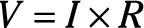In this formula, V stands for voltage (in volts), I stands for current (in amperes), and R stands for resistance (in ohms).

Here's an example of how to calculate voltage in a circuit with a lamp powered by the two AA cells. Suppose you already know that the resistance of the lamp is 12 Ω, and the current flowing through the lamp is 250 mA, which is the same as 0.25 A. Then, you can calculate the voltage as follows:

Ohm's law is incredibly useful because it lets you calculate an unknown voltage, current, or resistance. In short, if you know two of these three quantities you can calculate the third.

Go back (if you dare) to your high-school algebra class and remember that you can rearrange the terms in a simple formula such as Ohm's law to create other equivalent formulas. In particular:• If you don't know the voltage, you can calculate it by multiplying the current by the resistance.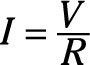• If you don't know the current, you can calculate it by dividing the voltage by the resistance.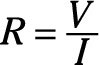• If you don't know the resistance, you can calculate it by dividing the voltage by the current.

To convince yourself that these formulas work, look again at the circuit with a lamp that has 12 Ω of resistance connected to two AA batteries for a total voltage of 3 V. Then you can calculate the current flowing through the lamp as follows: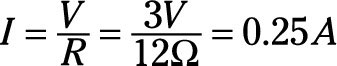If you know the battery voltage (3 V) and the current (250 mA, which is 0.25 A), you can calculate the resistance of the lamp like this: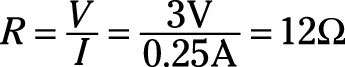Wasn't going back to high school algebra fun? Next thing you know, you're going to start looking for a prom date.

The most important thing to remember about Ohm's law is that you must always do the calculations in terms of volts, amperes, and ohms. For example, if you measure the current in milliamps (which you usually will in electronic circuits), you must convert the milliamps to amperes by dividing by 1,000. For example, 250 mA is 0.25 A.

Here are a few other things you should keep in mind concerning Ohm's law:

• Remember that the definition of one ohm is the amount of resistance that allows one ampere of current to flow when one volt of potential is applied to it? This definition is based on Ohm's law. If V is 1 and I is 1, then R must also be 1.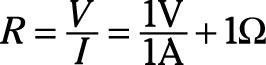• If you wonder why the symbols for voltage and resistance are V and R, which make perfect sense, but the symbol for current is I, which makes no sense, it has to do with history.

The unit of measure for current — the ampere — is named after André-Marie Ampère, a French physicist who was one of the pioneers of early electrical science.

The French word he used to describe the strength of an electric current was intensité – in English, intensity. Thus, amperage is a measure of the intensity of the current. Hence the letter I.

• In the interest of international cooperation, the term volt is named for the Italian scientist Alessandro Volta, who invented the first electric battery in 1800. (Actually, his full name was Count Alessandro Giuseppe Antonio Anastasio Volta.)